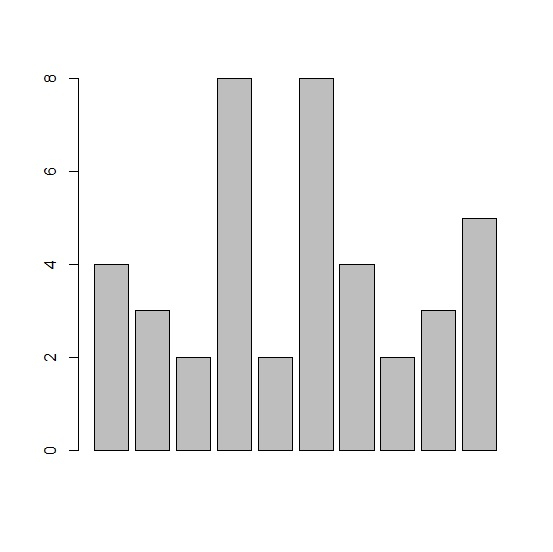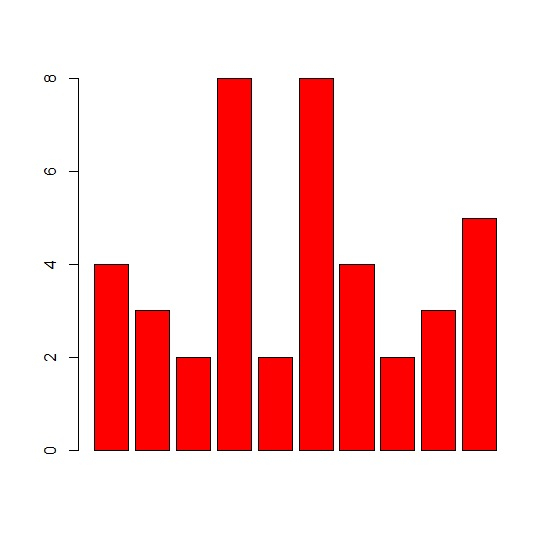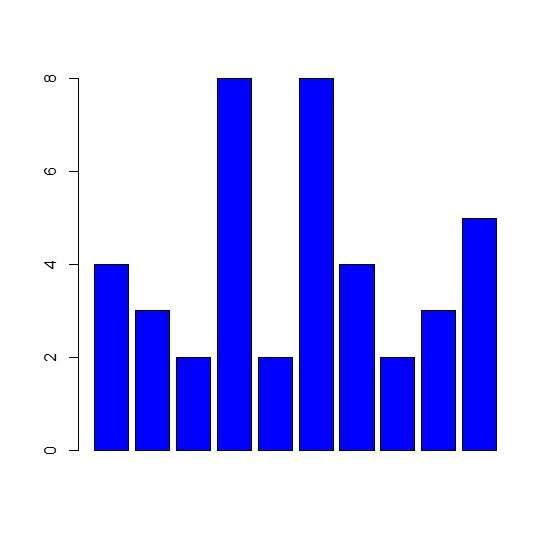# How to change the color of bars in base R barplot?

To change the color of bars in base R barplot, we can use col argument inside the barplot function.

For example, if we have a vector called V for which we want to create the barplot then we can use the command given below to get the bars in blue color −

barplot(V,col="blue")

Check out the below example to understand how it can be done.

## Example

To change the color of bars in base R barplot, use the code given below −

x<-rpois(10,5)
x

If you execute the above given code, it generates the following output −

 4 3 2 8 2 8 4 2 3 5

To change the color of bars in base R barplot, add the following code to the above code −

x<-rpois(10,5)
barplot(x)

## Output

If you execute all the above given snippets as a single program, it generates the following output −To change the color of bars in base R barplot, add the following code to the above code −

x<-rpois(10,5)
barplot(x,col="red")

## Output

If you execute all the above given snippets as a single program, it generates the following output −To change the color of bars in base R barplot, add the following code to the above code −

x<-rpois(10,5)
barplot(x,col="blue")

## Output

If you execute all the above given snippets as a single program, it generates the following output −